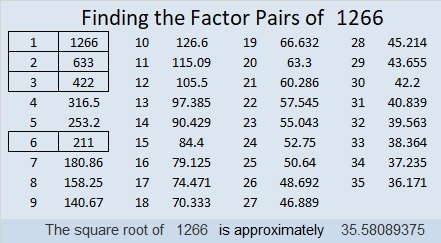# 1266 is a Centered Pentagonal Number

1266 is the 23rd centered pentagonal number because 5(22)(23)/2 + 1 = 1266. The graphic below shows 1266 tiny dots arranged into a pentagonal shape and that 1266 is one more than five times the 22nd triangular number.Here are some more facts about the number 1266:

• 1266 is a composite number.
• Prime factorization: 1266 = 2 × 3 × 211
• The exponents in the prime factorization are 1, 1, and 1. Adding one to each and multiplying we get (1 + 1)(1 + 1)(1 + 1) = 2 × 2 × 2 = 8. Therefore 1266 has exactly 8 factors.
• Factors of 1266: 1, 2, 3, 6, 211, 422, 633, 1266
• Factor pairs: 1266 = 1 × 1266, 2 × 633, 3 × 422, or 6 × 211
• 1266 has no square factors that allow its square root to be simplified. √1266 ≈ 35.580891266 is also the sum of ten consecutive prime numbers:
103 + 107 + 109 + 113 + 127 + 131 + 137 + 139 + 149 + 151 = 1266

This site uses Akismet to reduce spam. Learn how your comment data is processed.